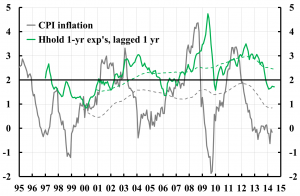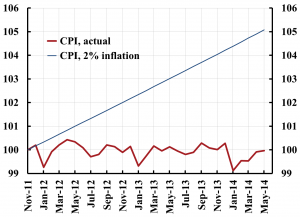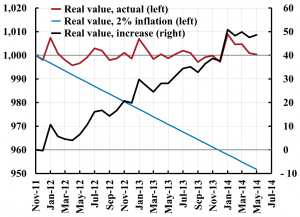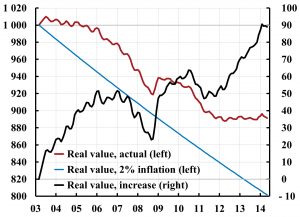# Why is household debt an additional reason for fulfilling the inflation target? (Updated June 2014)

Updated June 2014, with data of May 2014.

New Ekonomistas post. Here is an English translation.

Why is household debt an additional reason for the Riksbank to fulfill the inflation target? That is, in addition to the strong reason that the inflation target of 2 percent is how the legislated price-stability objective has been made operational. Well, this is because households expect inflation approximately equal to the target. If then inflation is allowed to fall below the target, the households suffer an unexpected and unwelcome capital loss on their debt, in the form of a higher real debt, compared if inflation had equaled the target. Currently, this capital loss is considerable, for instance, SEK 50 000 for each SEK million borrowed in the fall of 2011, and SEK 90 000 for each SEK million borrowed in the spring of 2003. The capital loss leads to an unexpected and unwelcome increase in households loan-to-value ratios and a reduction of their net worth, and the households’ resilience to disturbances is reduced, compared to if inflation had been 2 percent.

Figure 1 shows the actual annual CPI inflation rate and the inflation rate expected by the households. The difference between the grey and the green line thus shows the difference between actual inflation and the inflation expected by household, that is, the expectations they had a year earlier of inflation one year ahead. The dashed lines show five-year moving averages. We see that average inflation has undershot households’ average expectations since 1996, when the National Institute of Economic Research started to collect household expectations data.

The last observation in the figure is from May 2014. CPI inflation was then minus 0.2 percent. But households had expected inflation in May 2014 to be a about 1.7 percent. Actual inflation thus was about 2 percent lower than what households had expected.Figure 1. CPI inflation and household expectations a year earlier of inflation the next year, and 5-year moving averages. Sources: The National Institute of Economic Research and Statistics Sweden.

We also see in figure 1 that the actual inflation rate has been close to zero since the fall of 2012. That means that the price level now is about the same level as it was two and a half years ago.

Figure 2 shows the actual outcome of the CPI level from November 2011. The CPI has fluctuated somewhat up and down. In May 2014 it is on exactly the same level as it was in November 2011. The figure also shows what the outcome of the CPI would have been, if the inflation rate had been 2 percent. Then the CPI would have been 5 percent higher in May 2014. This difference has considerable consequences for the real value of household debt. I have discussed this in a previous Ekonomistas post (English translation here) in an op-ed in Expressen (in Swedish). Now I have discovered a new figure that can more clearly illustrate these consequences.Figure 2. Actual CPI inflation and CPI inflation if the inflation rate had been 2 percent since November 2011. Source: Statistics Sweden.

This new figure, figure 3, shows a time series of the real value of a loan of SEK 1 million that was taken out in November 2011 (red line, left scale, thousands of kronor). It has fluctuated up and down somewhat, but in May 2014 it is on exactly the same level, SEK 1 million, as in November 2011. The figure also shows a time series of the real value of the loan if the inflation rate had been 2 percent (blue line, left scale). Then the real value would have fallen by 2 percent each year and in November 2013 it would have been about SEK 950 000. This is the automatic real amortization that an inflation rate of 2 percent achieves. Finally, the figure shows a time series of the increase in the actual real value compared to the real value if the inflation rate had been 2 percent (black line, right scale, thousands of kronor), that is, the difference between the red and the blue lines. This difference grows from SEK 0 in November 2011 to SEK 50 000 in May 2014. For each borrowed SEK million, the borrower has thus lost SEK 50 000 in the last two and a half years, compared to if the inflation rate had been 2 percent. This is a substantial capital loss, especially in such a short time.Figure 3. The actual real value of a loan of SEK 1 million taken out in November 2011, the real value if the inflation rate had been 2 percent (both left scale, thousands of kronor), and the increase in the real value compared to if the inflation rate had been 2 percent (right scale, thousands of kronor).

Figure 4 shows the corresponding consequences for a loan of SEK 1 million taken out in March 2003, ten and a half years ago. The figure shows – as does figure 3 – a time series of the actual real value of this loan and the real value if the inflation rate had been 2 percent. It also shows the increase in the real value caused by the inflation rate being lower than 2 percent. We see that the actual real value of this loan is a full SEK 90 000 higher, compared to if the inflation rate had been 2 percent.Figure 4. The actual real value of a loan of SEK 1 million taken out in March 2003, the real value if the inflation rate had been 2 percent (both left scale, thousands of kronor), and the increase in the real value compared to if the inflation rate had been 2 percent (right scale, thousands of kronor).

For each borrowed SEK 1 million taken out in November 2011 and March 2003, respectively, the borrower has made a capital loss of SEK 50 000 and SEK 90 000, respectively. These are definitely sizeable amounts.

The value of the borrowers’ housing has increased in value during these years, both in nominal and real terms (see this updated post for details). But since housing is a real asset, for which the nominal value varies with the general price level, the nominal value of the housing becomes lower with lower inflation. The nominal value of the loan is fixed, independent of the inflation. Lower inflation hence leads to higher loan-to-value (LTV) ratios.

If, to start with, the real value of housing is assumed to be independent of inflation, the LTV ratio for the loan taken out in November 2011 or March 2003 has become 5 or 9 percent, respectively, higher than if the inflation rate had been 2 percent. However, probably the real value of housing is a bit lower than it would have been if the inflation rate had been 2 percent, since the tight monetary policy has implied higher interest rates, higher unemployment, and lower employment, compared to if the inflation rate had been equal to 2 percent. This means that the LTV ratios have increased more than 5 and 9 percent, respectively, compared to if the inflation rate had been equal to the inflation target.

If the low inflation had been expected, it would still have lead to the same less favorable outcome for the real value of a given loan (a slower real amortization of the loan). But the borrowers would have been compensated by lower nominal interest rates, since lower inflation expectations would have led to lower nominal interest rates. But this has not happened, since not only households but also money-market participants (read the banks, the lenders) and the other categories that are include in the Prospera survey have had inflation expectations close to the inflation target (see the discussion at the end of this previous Ekonomistas post, English translation) and this figure of 5-year moving averages of inflation expectations according to the Prospera survey).

In summary, an inflation rate, that is lower than the inflation target when household expect inflation in line with the target, does lead to unexpected and unwelcome capital losses on the households’ loans. With such low inflation as in Sweden, these capital losses are large. The mechanism is that the automatic amortization caused by 2 percent inflation is reduced or even eliminated. Since the low inflation is unexpected, the households have not been compensated by lower nominal interest rates. The result is that the households end up with higher real loans and higher LTV ratios, lower net worth, and lower net worth in relation to assets. The households’ resilience against disturbances becomes lower, compared to if the inflation rate is equal to 2 percent. All this provides an additional strong reason for the Riksbank to fulfill the inflation target.